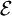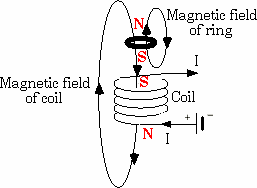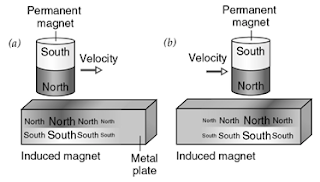### Magnetic Levitation

Maglev’s levitation is basically based on two simple and fundamental laws of electromagnetic.
• LENZ’S LAW

Faraday's law describes how an electric field can be induced by a changing magnetic flux, or in other words it describes about the induction of electric current.

It states that:
"The emf (electromagnetic force) induced in a circuit is directly proportional to the time rate of change of magnetic flux through the circuit."is the electromagnetic force (EMF) in volts
ΦB is the magnetic flux through the circuit (in Webers).

Faraday’s law simply says that, if a closed loop of wire is placed close to a permanent magnet, then electric current can be induced into the wire by moving either the magnet with respect to the coil or vice versa.
Hence it is the relative movement between the coil and the magnet that matters (change in flux cutting the loop).

When the magnet is moved toward the loop, the current induced flows in one direction, but when it is moved away, it flows in opposite direction, it indicates that the direction of the current depends on the time rate of change of the field, i.e. if the field is getting stronger or weaker as time progresses. The direction of induced current is further explained by Lenz’s law.LENZ’S LAW:

LENZ’S law describes about the direction of current being induced by magnetic field as described in faraday’s law.

It states that:
“Induced electromotive force generates a current, which flows in such direction as to induce a counter magnetic field that opposes the magnetic field generating the current”

The induced EMF creates a current that itself creates a secondary magnetic field. This secondary magnetic field also changes with time and thus creates a changing secondary magnetic flux. The secondary flux changes in such a way to oppose the change in flux creating the EMF.

To further understand, consider a coil and permanent magnet as shown in figure. No change in flux means no current induced. Now consider when the north pole of a permanent magnet is pushed into a loop (Fig b) the flux increases. An upwards secondary magnetic field is created that opposes the downward B-field of the magnet, and thus the current in loop must flow counterclockwise in order to create this secondary B-field.

When the magnet is removed from the loop (Fig c), the decreasing B-field in the loop creates a decreasing flux. To oppose this decrease, the current in the loop flows in such a way that tries to sustain the magnetic field. The current now has to flow clockwise in order to create a positive secondary flux that tries to counter acts the decreasing flux due to the with drawl of the permanent magnet.

How is magnetic levitation achieved?Magnetic levitation can be further understood by considering a current carrying coil. When current flows through the coil, it induces magnetic field. The change in magnetic field in the coil due to the change in current induces Eddy current in the metal ring, which induces magnetic field, as to oppose the field generating it. There repulsive force of the south-south pole (here) lifts the ring.

There are two types of magnetic levitation.
• Electromagnetic levitation (the ring example)
• Electrodynamics levitation.

The two figures as given further explain both type of magnetic levitations. Basically both employ same phenomenon, for levitation. In figure a, we have coil wrapped around the iron core, placed over a metal plate. Now when current flows through the coil, iron core is induced with the magnetic field.

This magnetic field as a result induces the magnetic field in the metal, but in opposite direction. Hence both fields repel each other and iron core is lifted upward. In figure b, we have a permanent magnet instead of iron core. When magnetic flux is changed by moving the magnet, it induces the magnetic field in the metal plate.

Thus both methods can be used to achieve levitation.Fig. (a)Fig (b)